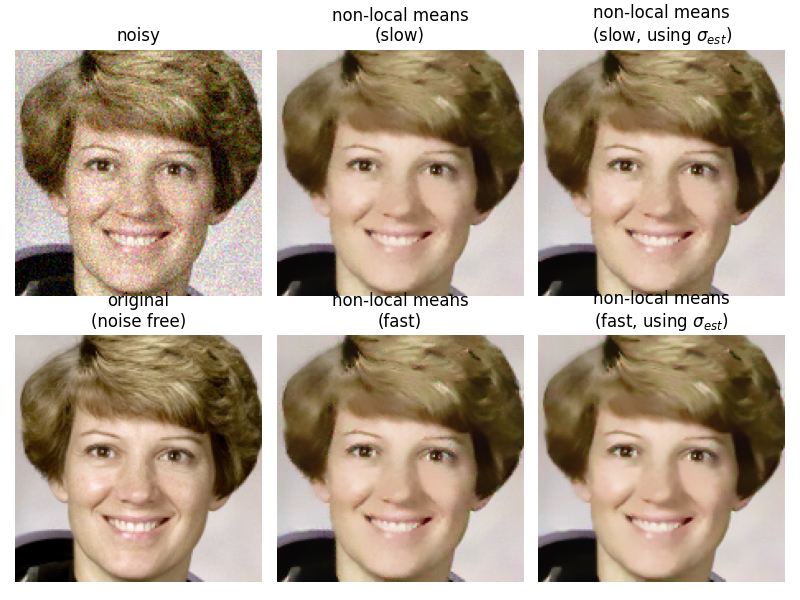# Non-local means denoising for preserving textures¶

In this example, we denoise a detail of the astronaut image using the non-local means filter. The non-local means algorithm replaces the value of a pixel by an average of a selection of other pixels values: small patches centered on the other pixels are compared to the patch centered on the pixel of interest, and the average is performed only for pixels that have patches close to the current patch. As a result, this algorithm can restore well textures, that would be blurred by other denoising algorithm.

When the fast_mode argument is False, a spatial Gaussian weighting is applied to the patches when computing patch distances. When fast_mode is True a faster algorithm employing uniform spatial weighting on the patches is applied.

For either of these cases, if the noise standard deviation, sigma, is provided, the expected noise variance is subtracted out when computing patch distances. This can lead to a modest improvement in image quality.

The estimate_sigma function can provide a good starting point for setting the h (and optionally, sigma) parameters for the non-local means algorithm. h is a constant that controls the decay in patch weights as a function of the distance between patches. Larger h allows more smoothing between disimilar patches.

In this demo, h, was hand-tuned to give the approximate best-case performance of each variant.estimated noise standard deviation = 0.07785763072771457
PSNR (noisy) = 22.18
PSNR (slow) = 29.29
PSNR (slow, using sigma) = 29.68
PSNR (fast) = 28.90
PSNR (fast, using sigma) = 29.28


import numpy as np
import matplotlib.pyplot as plt

from skimage import data, img_as_float
from skimage.restoration import denoise_nl_means, estimate_sigma
from skimage.metrics import peak_signal_noise_ratio
from skimage.util import random_noise

astro = img_as_float(data.astronaut())
astro = astro[30:180, 150:300]

sigma = 0.08
noisy = random_noise(astro, var=sigma**2)

# estimate the noise standard deviation from the noisy image
sigma_est = np.mean(estimate_sigma(noisy, channel_axis=-1))
print(f'estimated noise standard deviation = {sigma_est}')

patch_kw = dict(patch_size=5,      # 5x5 patches
patch_distance=6,  # 13x13 search area
channel_axis=-1)

# slow algorithm
denoise = denoise_nl_means(noisy, h=1.15 * sigma_est, fast_mode=False,
**patch_kw)

# slow algorithm, sigma provided
denoise2 = denoise_nl_means(noisy, h=0.8 * sigma_est, sigma=sigma_est,
fast_mode=False, **patch_kw)

# fast algorithm
denoise_fast = denoise_nl_means(noisy, h=0.8 * sigma_est, fast_mode=True,
**patch_kw)

# fast algorithm, sigma provided
denoise2_fast = denoise_nl_means(noisy, h=0.6 * sigma_est, sigma=sigma_est,
fast_mode=True, **patch_kw)

fig, ax = plt.subplots(nrows=2, ncols=3, figsize=(8, 6),
sharex=True, sharey=True)

ax[0, 0].imshow(noisy)
ax[0, 0].axis('off')
ax[0, 0].set_title('noisy')
ax[0, 1].imshow(denoise)
ax[0, 1].axis('off')
ax[0, 1].set_title('non-local means\n(slow)')
ax[0, 2].imshow(denoise2)
ax[0, 2].axis('off')
ax[0, 2].set_title('non-local means\n(slow, using $\\sigma_{est}$)')
ax[1, 0].imshow(astro)
ax[1, 0].axis('off')
ax[1, 0].set_title('original\n(noise free)')
ax[1, 1].imshow(denoise_fast)
ax[1, 1].axis('off')
ax[1, 1].set_title('non-local means\n(fast)')
ax[1, 2].imshow(denoise2_fast)
ax[1, 2].axis('off')
ax[1, 2].set_title('non-local means\n(fast, using $\\sigma_{est}$)')

fig.tight_layout()

# print PSNR metric for each case
psnr_noisy = peak_signal_noise_ratio(astro, noisy)
psnr = peak_signal_noise_ratio(astro, denoise)
psnr2 = peak_signal_noise_ratio(astro, denoise2)
psnr_fast = peak_signal_noise_ratio(astro, denoise_fast)
psnr2_fast = peak_signal_noise_ratio(astro, denoise2_fast)

print(f'PSNR (noisy) = {psnr_noisy:0.2f}')
print(f'PSNR (slow) = {psnr:0.2f}')
print(f'PSNR (slow, using sigma) = {psnr2:0.2f}')
print(f'PSNR (fast) = {psnr_fast:0.2f}')
print(f'PSNR (fast, using sigma) = {psnr2_fast:0.2f}')

plt.show()


Total running time of the script: ( 0 minutes 0.986 seconds)

Gallery generated by Sphinx-Gallery